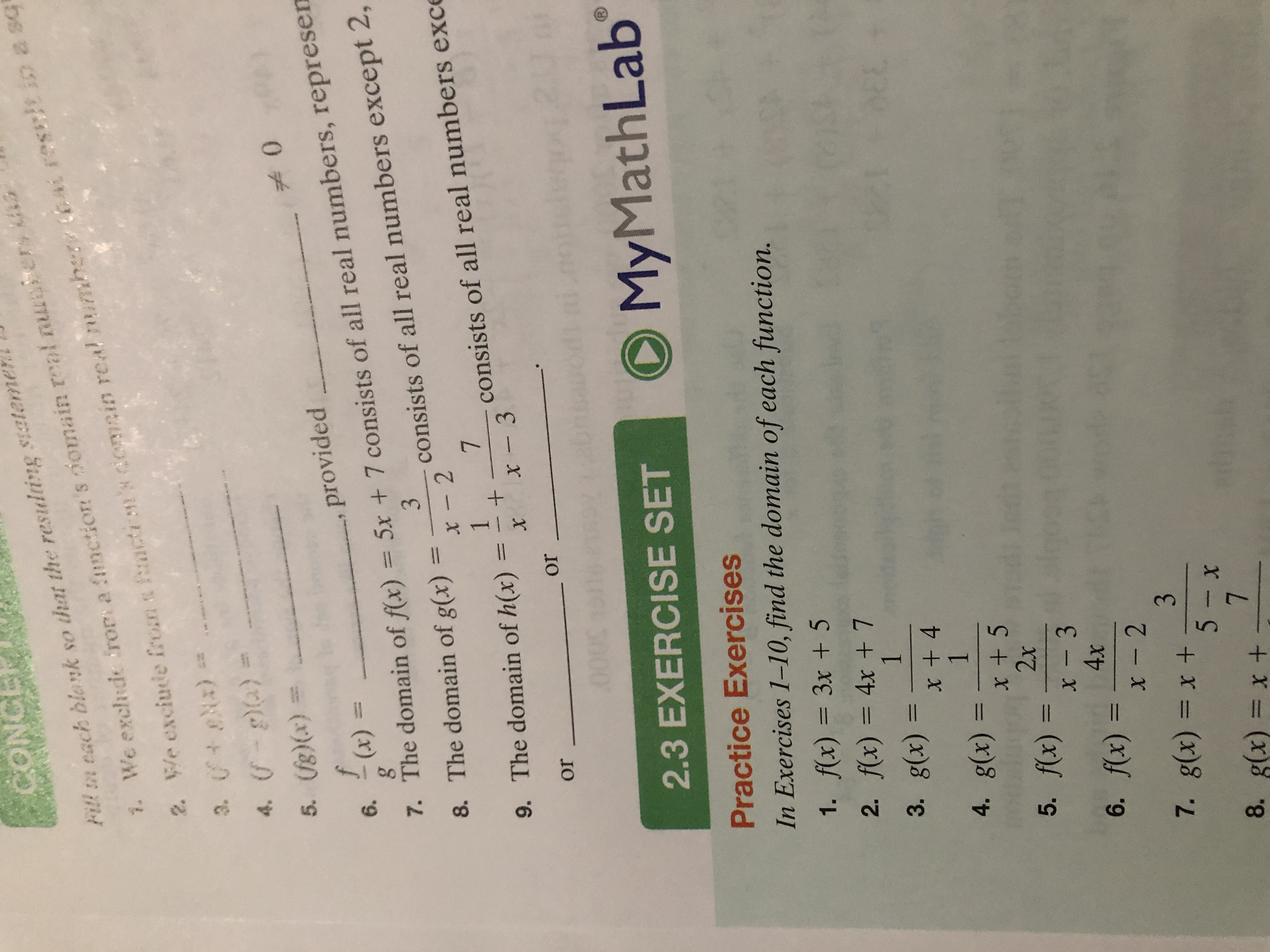CONCEFitl n each blank so that the restulting siatemebat rescicin a sq2 We exciute from a mctin's domcin real mumhe3. )4. )We exchde frors a function's omain real nuler7.5. (g)(x)0fprovided7. The domain of f(x) = 5x + 7 consists of all real numbers, represen8. The domain of g(x)(x)6.3consists of all real numbers except 2,x -271consists of all real numbers exce9. The domain of h(x)x- 3XororMath LabMy1)2.3 EXERCISE SETPractice ExercisesIn Exercises 1-10, find the domain of each function.1. f(x) = 3x +52. f(x) = 4x + 713. g(x)x + 414. g(x)x + 52x5. fx) =x - 34x6. fx)x- 237. g(x) = x +5- x78. g(x) = x ++

Question

How do you find the domain of each function for number onehelp_outlineImage TranscriptioncloseCONCE Fitl n each blank so that the restulting siateme bat rescicin a sq 2 We exciute from a mctin's domcin real mumhe 3. ) 4. ) We exchde frors a function's omain real nuler 7. 5. (g)(x) 0 f provided 7. The domain of f(x) = 5x + 7 consists of all real numbers, represen 8. The domain of g(x) (x) 6. 3 consists of all real numbers except 2, x -2 7 1 consists of all real numbers exce 9. The domain of h(x) x- 3 X or or Math Lab My 1) 2.3 EXERCISE SET Practice Exercises In Exercises 1-10, find the domain of each function. 1. f(x) = 3x +5 2. f(x) = 4x + 7 1 3. g(x) x + 4 1 4. g(x) x + 5 2x 5. fx) = x - 3 4x 6. fx) x- 2 3 7. g(x) = x + 5- x 7 8. g(x) = x + + fullscreen
Step 1

Since we only answer up to 3 sub-parts, we’ll answer the first 3. Please resubmit the question and specify the other subparts (up to 3) you’d like answered.

1. Given function is:

Domain of a function is defined as the set of the input values for which the output value is defined.

Here, the given function is defined for all real values of x, as the function is a polynomial.

Hence, the domain is R.

Since we only answer up to 3 sub-parts, we’ll answer the first 3. Please resubmit the question and specify the other subparts (up to 3) you’d like answered.

1. Given function is:

Domain of a function is defined as the set of the input values for which the output value is defined.

Here, the given function is defined for all real values of x, as the function is a polynomial.

Hence, the domain is R.

Step 2
1. Given function is:

Domain of a function is defined as the set of the input values for which the output value is defined.

Here, the given function is defined for all real values of x, as the function is a polynomial.

Hence, the domain is R.

Step 3
1. Given function is:

Domain of a function is defined as the set of the input values for which the output value is defined.

Here, the given function is always defined except when...

Want to see the full answer?

See Solution

Want to see this answer and more?

Our solutions are written by experts, many with advanced degrees, and available 24/7

See Solution
Tagged in

Algebra